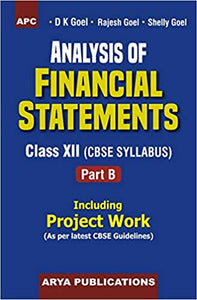# Analysis of Financial Statements Class XII, Part-B (Including Project Work)

Regular price
Rs. 463.12
Sale price
Rs. 463.12
Regular price
Rs. 475.00
Sold out
Unit price
per

The book has serveral unique features which make it distinct from other available books on this subject. Some of the special features of the book are:

Project Work as per latest CBSE Guidelines including Sample Questions for written test in projects.
Large number of questions based on Incomplete Information (i.e., missing figure questions) have been given.
The book also contains large number of One mark Questions.
A number of illustrations have been given in each chapter and these have been solved in such a simple manner that students can easily understand them.
Practical questions given at the end of each chapter are based on latest CBSE question papers and these are numbered according to the illustrations. Answers and hints to solve the questions have been given at the end of each questions, students can independently solve the practical questions.
The book contains objective-type, short-answer type and practical questions at the end of each chapter so that students may test their understanding of the chapter.
Weightage to each topic has been given according to the marks allotted to it by the CBSE.